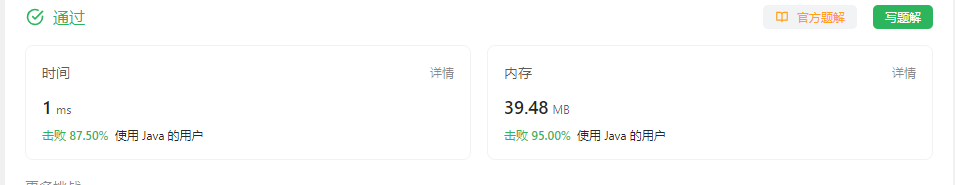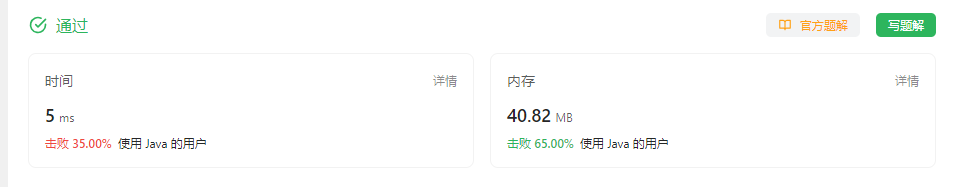• 累计撰写 158 篇文章
• 累计创建 26 个标签
• 累计收到 52 条评论

### 目 录CONTENT# 2562. 找出数组的串联值2023-10-12 / 0 评论 / 0 点赞 / 65 阅读 / 864 字 / 正在检测是否收录...

# 2562. 找出数组的串联值

• 例如，1549 的串联是 1549

nums串联值 最初等于 0 。执行下述操作直到 nums 变为空：

• 如果 nums 中存在不止一个数字，分别选中 nums 中的第一个元素和最后一个元素，将二者串联得到的值加到 nums串联值 上，然后从 nums 中删除第一个和最后一个元素。
• 如果仅存在一个元素，则将该元素的值加到 nums 的串联值上，然后删除这个元素。

输入：nums = [7,52,2,4]

- 在第一步操作中：

- 在第二步操作中：



输入：nums = [5,14,13,8,12]

- 在第一步操作中：

- 在第二步操作中：

- 在第三步操作中：
nums 只有一个元素，所以我们选中 13 并将其加到串联值上，所以串联值等于 673 。



### 题解

class Solution {
public long findTheArrayConcVal(int[] nums) {
if (nums.length == 1) {
return nums;
}

int i = 0, j = nums.length - 1;
long sum = 0;
while (i < j) {

int n = nums[j];
nums[i] *= 10;
while (n / 10 != 0) {
nums[i] *= 10;
n /= 10;
}
sum += nums[i] + nums[j];
i ++;
j --;
}
if (nums.length % 2 != 0) {
sum += nums[nums.length / 2];
}
return sum;
}
}


#### 优化

class Solution {
public long findTheArrayConcVal(int[] nums) {

int i = 0, j = nums.length - 1;
long sum = 0;
while (i <= j) {
if (i == j) {
return sum + nums[i];
} else {
int n = nums[j];
nums[i] *= 10;
while (n / 10 != 0) {
nums[i] *= 10;
n /= 10;
}
sum += nums[i] + nums[j];
i ++;
j --;
}
}
return sum;
}
}#### 偷懒写法

class Solution {
public long findTheArrayConcVal(int[] nums) {
long sum = 0;
for (int i = 0, j = nums.length - 1 ; i <= j; i++, j--) {
if (i == j) {
sum += nums[i];
} else {
sum += Integer.valueOf(String.valueOf(nums[i]) + String.valueOf(nums[j]));
}
}
return sum;
}
}0